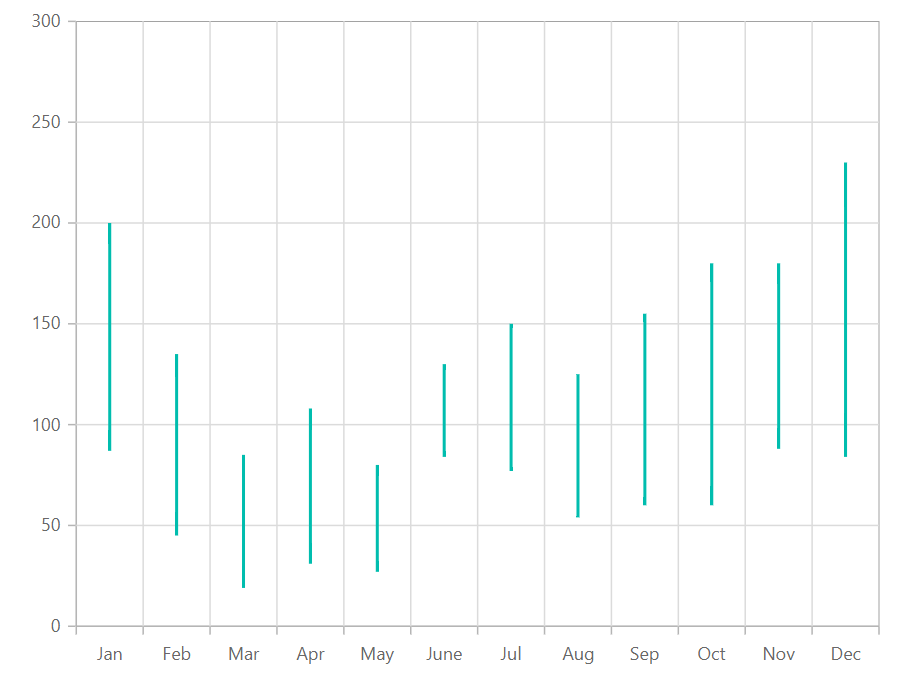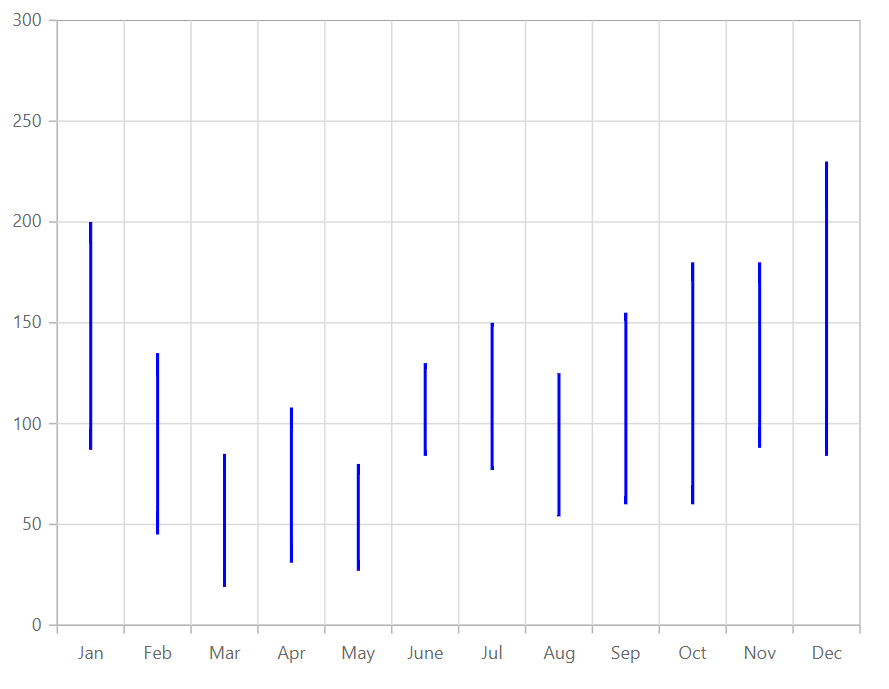Search results

# Hilo in Blazor Chart component

08 Apr 2021 / 2 minutes to read

Hilo Series illustrates the price movements in stock using the high and low values. To render a Hilo series, use series `Type` as Hilo.

Hilo series requires 3 fields (x, high and low) to show the high and low price in the stock.

hilo.razor
``````@using Syncfusion.Blazor.Charts

<SfChart>
<ChartPrimaryXAxis ValueType="Syncfusion.Blazor.Charts.ValueType.Category"/>

<ChartSeriesCollection>
<ChartSeries DataSource="@StockDetails" XName="X" High="High" Low="Low" Type="ChartSeriesType.Hilo">
</ChartSeries>
</ChartSeriesCollection>
</SfChart>

@code{
public class Data
{
public string X { get; set; }
public double Y { get; set; }
public double High { get; set; }
public double Low { get; set; }
}

public List<Data> StockDetails = new List<Data> {
new Data{ X= "Jan", Low= 87, High= 200 },
new Data{ X= "Feb", Low= 45, High= 135 },
new Data{ X= "Mar", Low= 19, High= 85 },
new Data{ X= "Apr", Low= 31, High= 108 },
new Data{ X= "May", Low= 27, High= 80 },
new Data{ X= "June",Low= 84, High= 130 },
new Data{ X= "Jul", Low= 77, High=150 },
new Data{ X= "Aug", Low= 54, High= 125 },
new Data{ X= "Sep", Low= 60, High= 155 },
new Data{ X= "Oct", Low= 60, High= 180 },
new Data{ X= "Nov", Low= 88, High= 180 },
new Data{ X= "Dec", Low= 84, High= 230 }
};
}``````Note: You can refer to our `Blazor Hilo Chart` feature tour page to know about its other groundbreaking feature representations. You can also explore our `Blazor Hilo Chart example` to knows how to render and configure the Hilo type series.

## Customization

You can use the following properties to customize the hilo series.

``````@using Syncfusion.Blazor.Charts

<SfChart>
<ChartPrimaryXAxis ValueType="Syncfusion.Blazor.Charts.ValueType.Category" />

<ChartSeriesCollection>
<ChartSeries DataSource="@StockDetails" XName="X" High="High" Low="Low" Fill="blue" Type="ChartSeriesType.Hilo">
</ChartSeries>
</ChartSeriesCollection>
</SfChart>

@code{
public class Data
{
public string X { get; set; }
public double Y { get; set; }
public double High { get; set; }
public double Low { get; set; }
}

public List<Data> StockDetails = new List<Data> {
new Data{ X= "Jan", Low= 87, High= 200 },
new Data{ X= "Feb", Low= 45, High= 135 },
new Data{ X= "Mar", Low= 19, High= 85 },
new Data{ X= "Apr", Low= 31, High= 108 },
new Data{ X= "May", Low= 27, High= 80 },
new Data{ X= "June",Low= 84, High= 130 },
new Data{ X= "Jul", Low= 77, High=150 },
new Data{ X= "Aug", Low= 54, High= 125 },
new Data{ X= "Sep", Low= 60, High= 155 },
new Data{ X= "Oct", Low= 60, High= 180 },
new Data{ X= "Nov", Low= 88, High= 180 },
new Data{ X= "Dec", Low= 84, High= 230 }
};
}``````Note: You can refer to our `Blazor Charts` feature tour page for its groundbreaking feature representations. You can also explore our `Blazor Chart example` to knows various chart types and how to represent time-dependent data, showing trends in data at equal intervals.

Contents
Contents